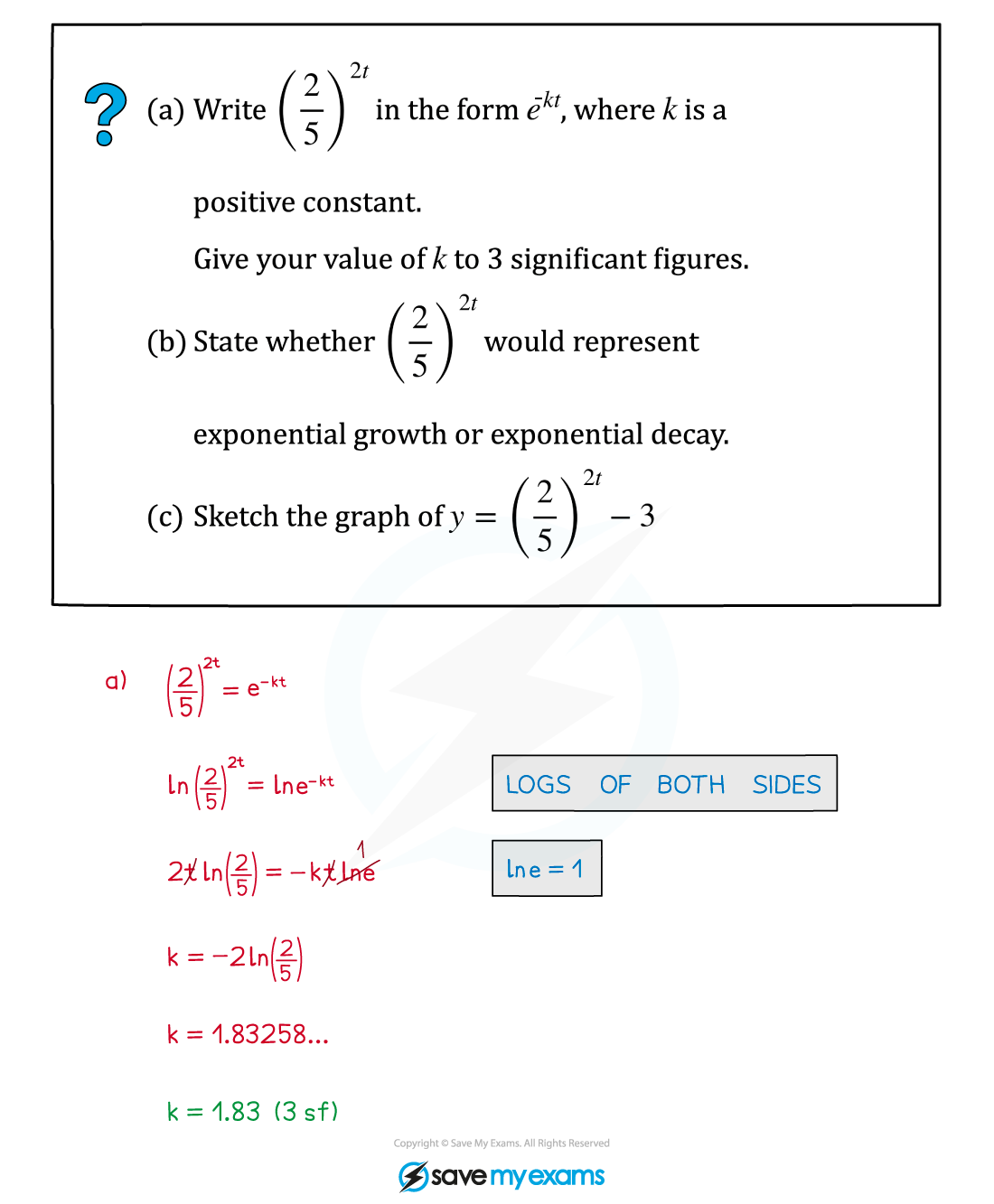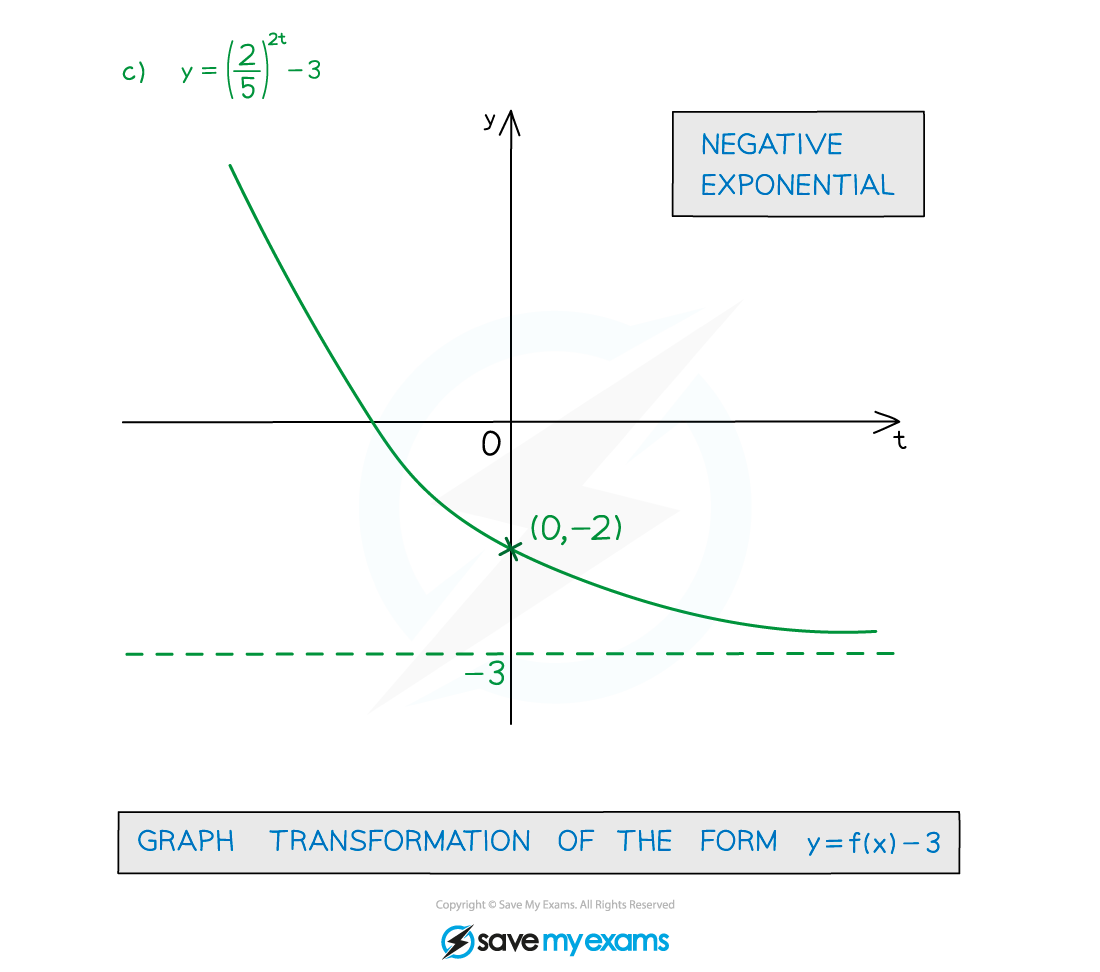# AQA A Level Maths: Pure复习笔记6.3.1 Exponential Growth & Decay

### Exponential Growth & Decay

#### What are exponential growth and exponential decay?

• y = Aekt is exponential growth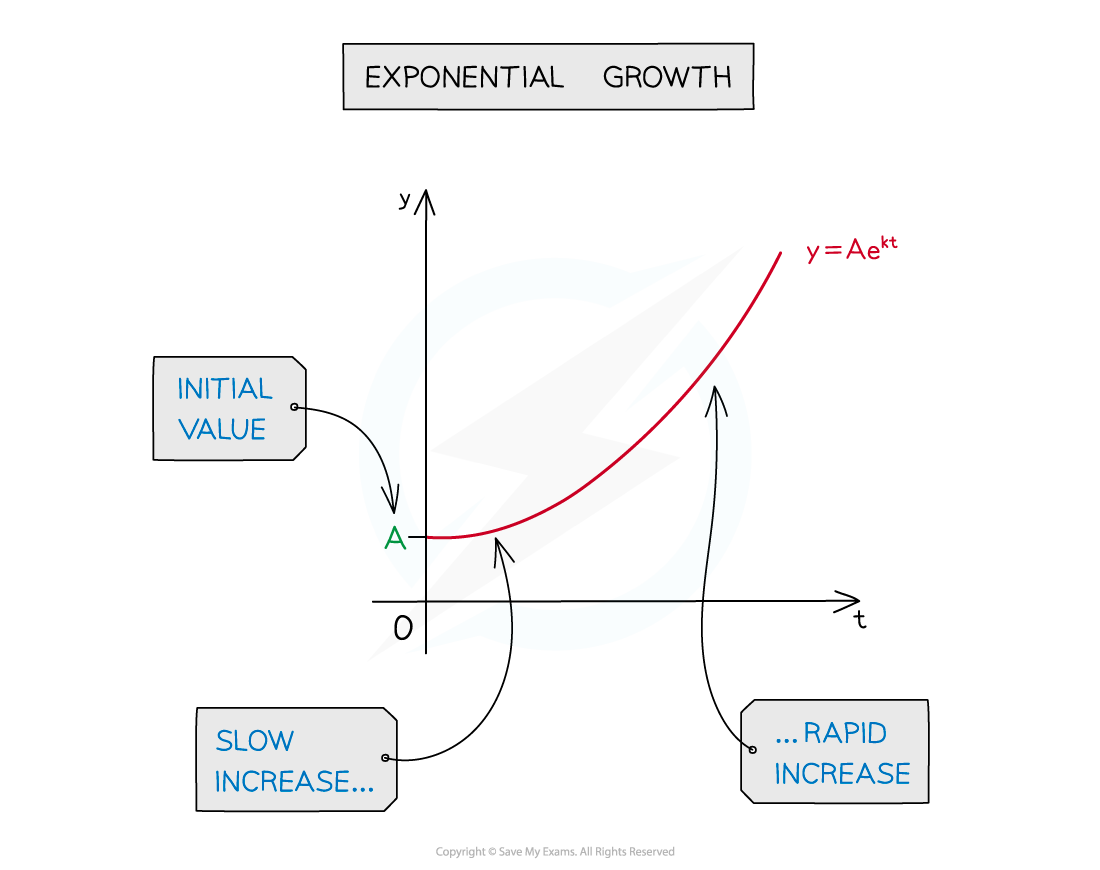• y = Ae-kt is exponential decay• k > 0 so plus or minus sign in equation immediately indicates growth or decay
• A is the starting value or initial value, when t = 0
• t is often used, rather than x, as many models are time-dependent

#### Is e always used in exponential growth and decay?

• Using e makes the maths easier
• With the proper choice of k, all exponential functions of the form acan be written in the form ekx
• a= ekx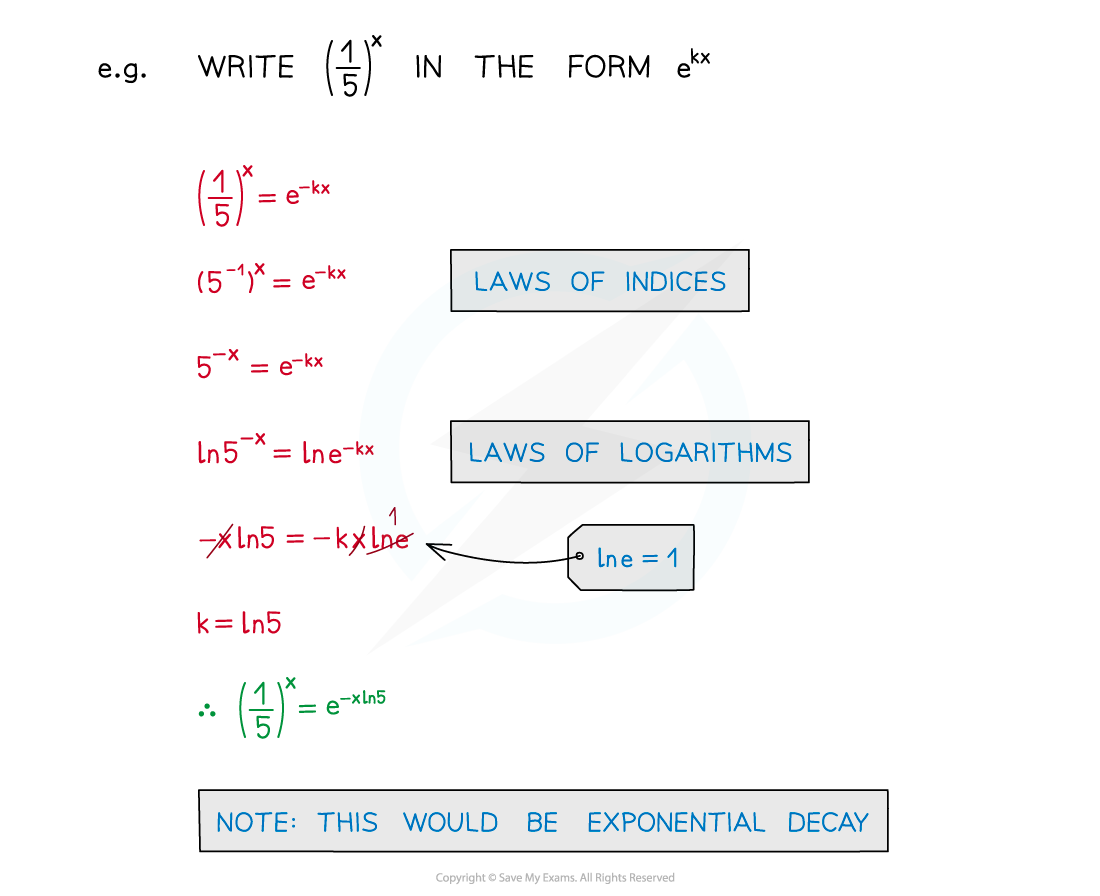#### Will logarithms or ln be involved?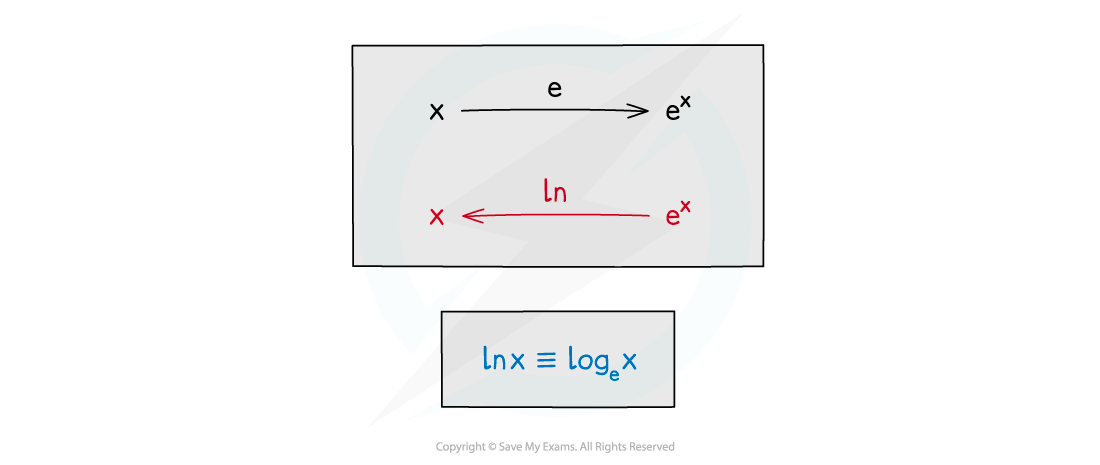• ln is the inverse of e• The graph of y = ln x is the reflection of the graph of y = ex in the line y = x

#### Worked Example